Select PageIn our previous article about the derivation of the Hebrew Alphabet we showed how the Alef-bet, like the mathematical constant Phi(φ), is based on the Principle of One.  It is all about frequency and vibration and what that means for us as it filters into our dimensional plane, especially through the Hebrew Alef-bet, the cosmic interface to the language of the upper dimensions.

There is a Path of One within the Torah and Creation, so named because it is solely based on 13 and 13 is the numerical value of Echad (אחד), meaning One, as in “G-d is One,” and because all the frequencies of the Alef-bet are derived from 1.313 and its intersection with the field of Phi(φ). The Path’s derivations are strictly numeric and geometric, yet they all integrate with the specific Names of G-d within the Torah, and what those Names connect with beyond the confines of physicality.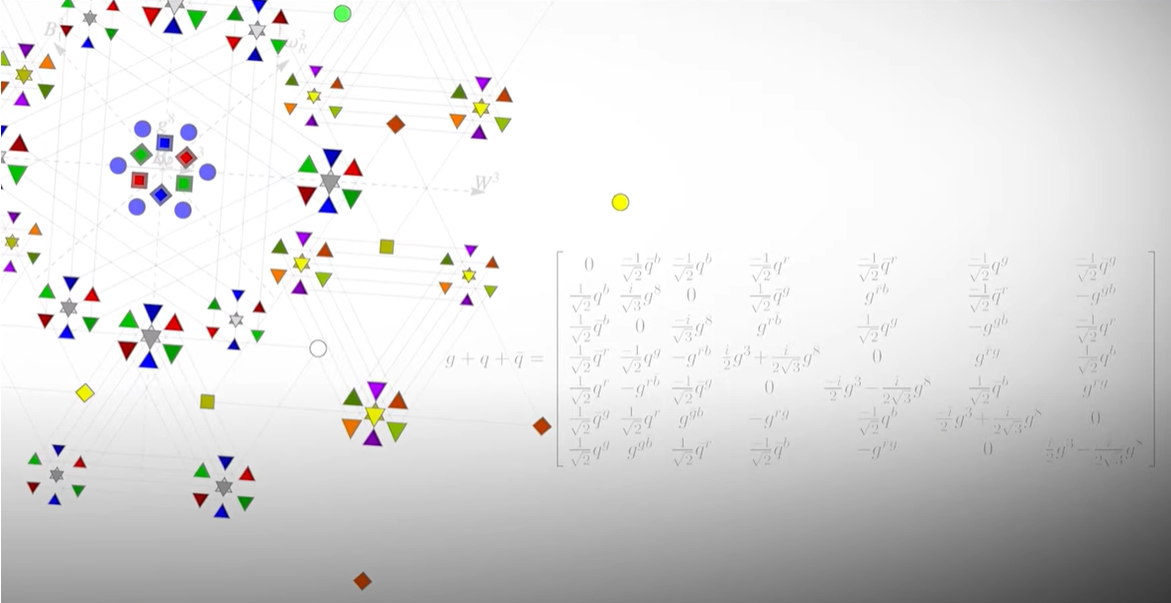Physicality is built on geometry. That is the nature of 3-dimensional structure. That is how we understand our world and our being, our existence. The three main geometric shapes filtered through the Torah into our realm are the sphere and circle; the cube, including an assortment of hypercubes; and the triangle. While the symmetries of the sphere and the cube are mostly radial in nature, that of the triangle is more vertical and power driven. The triangle begins as a point and expands and builds as it grows deeper and richer.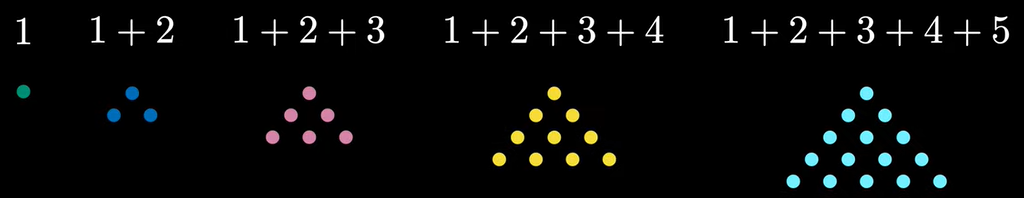The Names of the Creator and the basic concepts of the cosmos or greater universe are schematically conveyed to us, or made transformative to us, through these simple but hidden geometries.

The concept behind Triangular Numbers is that nothing is ever lost and that everything that ever existed prior is still there whether we see it or not. For example, everything that got us to this moment is our lives is still present in this very moment and our present actions are the results of everything that happened unto this moment. This concept is so important for the Torah to convey to us and in the operation of the greater universe that the value of the Torah’s first verse, 2701, is the sum of the first 73 positive integers, the 73rd Triangular Number; the sum of the last 2 words of that 7-word verse is the sum of the first 37 integers, 703, the 37th  Triangular Number; while the sum of the first 5 words is 1998 or 3 times the 36th Triangular Number; and the first 8 words or first 33 letters is 3003, the 77th Triangular Number, like the 77 times the Name Adonai (אדני) appears in the Torah. Moreover, the value for that first verse, 2701, equals (37 x 73), and represents the value and the ordinal or inner value of the word, Chochma, meaning Wisdom, the 2nd highest sefira or dimension.

The Path of One, naturally begins with One (1) and grows in a series of numeric triangles that build upon each other and that each have 13 levels.

That Path of One is (113914551820). Its first special property is its connection to the Cosmic Singularity of 42, one of the deepest concepts in cosmology and metaphysics that we have literally written books about and that we will explore profoundly later in this series.  For now, please just know that 42 was a pure concept long before it was a number, which is why it plays such a prominent role in the Torah and why the Israelites had to make 42 journeys to cross the wilderness to enter the promised land.

Like the 5 Books of Moses, the Path of One has 5 components that sum to (1 + 13 + 91 + 455 + 1820) = 2380. This simple equation expresses the expansive aspect of the two archetype forces/fields of Jacob (182) and of Rachel (238), whereby, like the love between the Biblical couple (182 + 238) that equals 420, the Path of One (1820 + 2380) = 4200 as well.  And while this itself represents the Cosmic Singularity 42, the inverse of the equation or 1/2380 = 42 cements it.

Like the Alef-bet that is based on 1.313x and the Torah that was gifted to Man in 1313 BCE at Mt Sinai (130), the Path of One is also the Path of Love, Ahava (אהבה) and Oneness, Echad (אחד) both of numerical value 13.

It starts with One. Then if we sum up the first 13 integers (1 +2 + 3…13), we get the 13th Triangular Number 91.

 1 1 1 1 2 3 4 5 3 6 10 15 4 10 20 35 5 15 35 70 6 21 56 126 7 28 84 210 8 36 120 330 9 45 165 495 10 55 220 715 11 66 286 1001 12 78 364 1365 13 91 455 1820 91 455 1820 6188

If we then sum up the cumulative values of those 13 integers, in other words, the first 13 Triangular Numbers we get 455, the 3rd value in the Path of One.

Next, when we sum up the cumulative values of those 13 Triangular Numbers to get 1820, the 4th and final value in the Path of One, we reach the value directly corresponding to the 1820 YHVH (יהוה) in the Torah.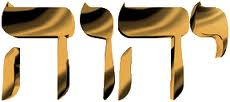As explained in our last article about the origin of the Hebrew Alef-bet, the derivation of the 22 Hebrew letters came from the One (1) and from the 22 iterations that it takes to descend 1 order of magnitude by 10% each time. In other words, from 1000 to 100 or 100 to 10 or 10 to 1, multiplying by 90% or 0.90 each time.

We know that this was not a lucky guess by a primitive people because the nominative counting system, namely those assigned 22 bundles or values, like the 90% ratios, are also distributed in sets of 9, forming groups of ascending orders of magnitude 1 – 10, 10 – 100 and 100 – 1000.

 10 1 9 2 8.1 3 7.29 4 6.561 5 5.9049 6 5.31441 7 4.78297 8 4.30467 9 3.8742 10 3.48678 11 3.13811 12 2.8243 13 2.54187 14 2.28768 15 2.05891 16 1.85302 17 1.66772 18 1.50095 19 1.35085 20 1.21577 21 1.09419 22 0.984771 91.1371

As we can see, the sum of those 22 iterations based on 10, which is ostensibly 1 because the same thing happens if we start at 1, is 91.13706188. Astoundingly, this sum aligns incredibly well with the Path of One in that the sum of the 13 integers is 91; the 1st order of 13 is (1 x 13) = 13; and the sum of the cumulative values of those 13 cumulative values of the 13 Triangular Numbers that equal 1820 is 6188, as in 91.13706188.

What is even more astonishing though is that the sum of the 22 iterations based on One, 91.13706188, is 91.0 plus the Fine Structure Constant, 1/137.06188, off from what that number is believed to be by a mere .01889%. This is incredibly close, considering it is usually abbreviated by scientists as 1/137 and considered to be one of the most important constants in the universe, and one that all our physics seems to hinge on.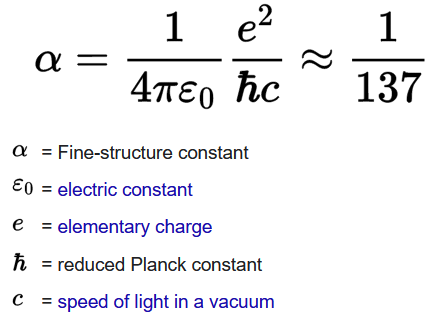Interestingly, the Fine Structure Constant is always defined as dimension 1. The Fine Structure Constant plays innumerable roles in physics and is most known as the “coupling constant” or the measure of the strength of the electromagnetic force that governs how electrically charged elementary particles (i.e., electron, muon) and light (photons) interact. It is equally important as a field for those forward thinkers who envision the universe as pools of pressure modalities and mediations that present as energy in lieu of particles.

In the sum 91.13706188 we can possibly see 1/Phi(φ) or .618 again, while we must keep in mind that the number from the Path of One, 1820, has 22 factors, not including itself and 1, which circles us back to the 22 letters and 22 iterations that gave us 91.13706188.

While the Creator of the Alef-bet and the Torah made sure that the 22 letters, our interface to the Torah, would synch flawlessly and align harmonically with all the same inherent constants or modality fields that the physical universe is constructed with, and upon which all existence is predicated, such as Phi(φ), Pi (π), and the Fine Structure Constant, He also aligned the Path of One and its few elements with the Names of G-d found in the Torah.

### The Path of the Names

We have already spoken about the 1820 Names YHVH (יהוה), each of numerical value 26, in the Torah and shown the relationships between 2380 and 1820 to 1/42 and 4200, so it cannot be coincidental that the 42nd set of the 91 sets of doubled words in the Torah is (יהוה) (יהוה), as in the 2626 value of the set of 11 Odd Names of the 22 Names of the 22 Letters that are directly related to Phi(φ) in 5 different ways. Or that the 420th Name YHVH (יהוה) in the Torah begins the 13 verses of the 10 Commandments at Mt Sinai, whose numerical value is 130. Or that the Name Yehuda (יהודה), which contains the YHVH (יהוה) in order and is one of the 12 Tribes and the progenitor of King David, is found 42 times in the Torah.

The value 91 itself, the 13th Triangular Number, represents the unification of the YHVH (יהוה), 26, and its pronounceable counterpart Adonai (אדני), 65, of which there are exactly 91 occurrences in the Torah, like the 91 paragraphs in the Torah’s first Book. Their simple gematria equation is (26 + 65) = 91, which is also the numerical value of Amen (אמן). Said after a blessing to complete and empower it, the word Amen (אמן) itself is found only 15 times in the Torah. The first time we encountered the word Amen is as one of those 91 doubled words (אמן) (אמן) in portion Naso 5:22, giving the two of them a value of 182. The next 11 times it is found at the end of 11 consecutive verses in portion Ki Tavo 27:15-26, which aligns with the 1526 exact number of pure YHVH (יהוה) that have no prefixes, out of the 1820 total in the Torah. The final time the word Amen (אמן) occurs is in the prophetic song Hazeinu 32:20 where the verse ends Amen Bam(אמן־בם) or “Amen 42.”

As for the cumulative value of the first 13 Triangular Numbers, or 455, it is the value of the 3 Aspects, or spelled-out Names of the letters, of the Name Ehyeh (אהיה), the highest Name of the Creator used in the Torah.

## אלף־הא־יוד־הא

As the adage goes, less is more and the Name Ehyeh (אהיה), is found only 7 times in the Torah, leading to the equation 1820/7 = 260, as in the value of the YHVH (יהוה) times 10. Equations are the universe’s way of illustrating the relationships between its components and it concepts. They often illuminate the intersection of the cosmic networks that we would otherwise miss.

Combined, the total gematria value of 1820 YHVH (יהוה), which is (1820 x 26) and the 7 Ehyeh (אהיה) or (7 x 21) when divided by the Phi (φ) angle (137.5o) is ((1820 x 26) + (7 x 21))/137.5  = 345.214, represents the Spirit/Soul, Ruach (רוח) of numerical value 214, of Moses (משה), of numerical value 345 that runs through the Torah.

The 4 Names, Ehyeh (אהיה), YHVH (יהוה), Elohim (אלהים), and Adonai (אדני) are found 7, 1820, 313, and 91 times in the Torah respectively, leading to the equation of the 4 Names: (7 x 21) + (1820 x 26) + (313 x 86) + (91 x 65) = 80,300. At first this result seems promising yet devoid of meaning to us. That is until we realize that there are 304,805 letters in the Torah and of those 304,805 letters, 4805 of them are the letter Pe (פ) of numerical value 80, leaving exactly 300,000 other letters, thus we see the intersection of the 80,300-valuation of the set of 4 Names and the 80 plus 300,000 letters of the Torah.

Moreover, those 1820 YHVH (יהוה) are constructed out of the exact 90,100 letters Yuds(י), Heis (ה), and Vavs (ו) in the Torah, as in 90100, the 424th Triangular Number. The number and concept of 424 is unique and special because it is the numerical value of Moshiach Ben David, known as the Messiah in English.  Let us think about that for a moment: about one-third of all the letters in the Torah form a triangle or alpha-numeric pyramid of exactly 424 levels, all constructed out of variations of the Name of G-d YHVH (יהוה).

There are 3 phases of Names of the Creator in the Torah. In the first phase there are 35 Names Elohim (אלהים), 32 of which are in the Torah’s first Chapter about the first 6 Days of Creation.  Then in Genesis verse 2:4, the Name Elohim (אלהים) get replaced by YHVH-Elohim (אלהיםיהוה). That continues for a total of 20 times in the next 2 chapters until it switches for a final time to YHVH (יהוה). It is Eve (Chava) who is the first person to utter the Name YHVH (יהוה) in Genesis 4:1. While those 35 times align with the 35 times that the word Bam (בם), the signature initials of 42, is used, they sum with the next phase of 20 YHVH-Elohim (אלהיםיהוה) to equal (35 + 20) = 55 times that the Names Elohim (אלהים) and YHVH-Elohim (אלהיםיהוה) are utilized in the first 2 phases, leaving exactly (182020) = 1800 times that the Name YHVH (יהוה) is utilized in the 3rd phase.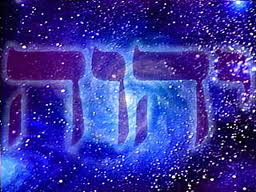The square root of 1800 is 42.426…, as in the numerical values of Moshiach Ben David (424) and the 26-value of the YHVH (יהוה) constructed out of the precise 90,100 letters Yuds (י), Heis (ה), and Vavs (ו) in the Torah.

That split into 55 and 1800 is far from arbitrary. We will not go into right now the esoteric properties of, or the importance of 55 being the entrance to the Holy Names in the Bible, other than to say that it relates to the 10 sefirot (dimensions) of the Tree-of-Life, and the 2 letters Hei (הה) in the YHVH (יהוה).  We have covered that extensively in previous articles/books and will do so again in due time.

The 4 letters in the YHVH (יהוה) are found as a set in any order within any word in the Torah exactly the 2115 times and (2115 x 26) = (55,00010).  The number and concept of 55 is entwined with the concept of 10 and integrated into its geometry. As we can see, the sum of the first 10 integers (1 + 2 + 3…10) equals 55, and they form a 4-tier Triangle, the 4th Triangular Number, as 1 + (2 + 3) + (4 + 5 + 6) + (7 +8 + 9 + 10) = 55.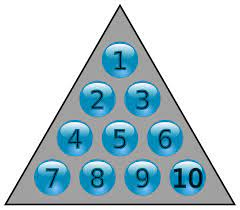That 55 total value of the pyramid of 10, subdivides into a sub-triangle or pyramid of (2 + 4 + 5 + 7 + 8 + 9) = 35 and the remaining right-side edge that is composed of the 1st, 2nd, 3rd, and 4th Triangular Numbers (13610) that sum to 20. We can now understand how the scaffold of the Names of the Creator in the Torah align perfectly with the geometry of the Triangular Numbers, including that the 4 tiers and 4th Triangular Number correspond to the 4 Letters in the Name YHVH (יהוה).

The 2 complementary sections of the pyramid of 10 that correspond to the first 2 phases of the Names of the Creator in the Torah act as a capstone or pyramidion to the rest of the Names in the Torah. It is also a 4-tier capstone to the other 420 tiers of the 90,100 Yuds(י), Heis(ה), and Vavs (ו) in the Torah. We now have a better understanding why the 10 Commandments begin with the 420th YHVH (יהוה).Moreover, the flip side triangle (3 – 5 – 6 – 8 – 9 – 10) has a total value of 41, as in the ordinal value of each of those 35 Elohim (אלהים), starting with the Torah’s 3rd word. If we sum up all 10 sub-Triangles in this 4th Triangular Number we get (55 + 35 + 41…6) plus 4 for the 4 tiers of the 4th Triangular Number equals 253, which is the 22nd Triangular Number, as in the 22 letters of the Alef-bet.

It is also as in the 22 iterations that descend from 100, by increments of 90%, as in 90,100. Every Number and letter in the Torah serve in multiple equations and networks at once.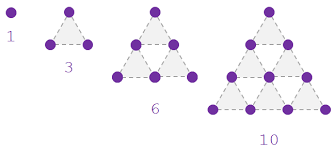As we get deeper into the geometries of the Torah and the cosmos with which it aligns, other properties other than their vertices become more significant likes their edges and faces. In the case of this Triangle of 10, there are 18 edges and like 1800, the square root of 18 is 4.2426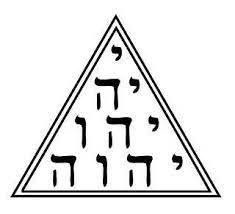When the Name YHVH (יהוה) replaces the 10 positions in the 4th Triangular Number, the 10 regressively expanded Letters Y-YH-YHV-YHVH (י־יה־יהו־יהוה) sum to 72, which is an entirely other long topic of discussion for another day. Nonetheless, the also correspond to the 10 letters in the 4 Names of the Letters YHVH (יהוה) or YVD-HY-VYV-HY (יוד־הי־ויו־הי) that also sum to 72.

Meanwhile, the 9 facets or small triangles (1 – 2 – 3, 2 – 4 – 5…) in the numerical version equal 144, which is twice 72, but is also the 12th Fibonacci Number, which is curious because 55 is the 10th Fibonacci Number. Since we have acknowledged that 55 is associated with the first 2 phases and the first 55 Names of the Creator in the Bible, we should also note that there are exactly 144 YHVH (יהוה) without prefixes in the Book of Genesis.

The YHVH (יהוה) equivalent for the 9 facets is 181, the 42nd Prime Number, and the sum of the first 42 digits in the set of all Prime Numbers.

Everything always derives from the Source, whether through 1 or 42. In this case, we can see that the value of the 10 positions or vertices, 72, plus the sum for the 9 facets is (72 + 181) = 253, the 22nd Triangular Number, the sum of the 22 ordinal values of the 22 letters of the Alef-bet.

Like the Cosmos on which it reflects, everything in the Torah is networked together and fractally faceted so that no matter what aspect we latch onto we can always access the whole.

### The Taxicab Number

Mathematicians will recognize the number 1729 as the Taxicab Number; nevertheless, there are 1729 words/Names in the Torah with the value 26, which is (182091). It is all about connecting to the One, and love, which is why the numerical value of the famous phrase from Leviticus 19:18 that is said to be at the heart of the Torah, “but thou shalt love thy neighbor as thyself (וְאָהַבְתָּ לְרֵעֲךָ כָּמוֹךָ)” has the gematria 820, leaving 1000 as Alef (One).

The entire anti-religious, anti-Creator narrative that has been built up in man’s belief system is that our religions are based on a mere collection of myths and stories borrowed from Babylonia and other pagan faiths and falsely believed to have been written by a Divine being. Yet the Truth is that not a single letter or number in the Torah is out of place, is without deep powerful meaning and significance, nor has even been changed.  Yes, the descendants of those pagan faiths have slipped in other versions over time and have tried to trick us into believing that translations into Greek or Aramean are more accurate, and even tried to convince us that the interpretations are more important than the text, but the Creator has made sure that the Torah survived intact, unadulterated for the last 3330 years. This is why ever since the Torah was gifted to us back on Mt Sinai, the 420th YHVH (יהוה) has marked the start of the 10 Commandments, and that this is also at the 363rd YHVH (יהוה) without a prefix, as in the numerical value of H’Moshiach, “The Messiah.”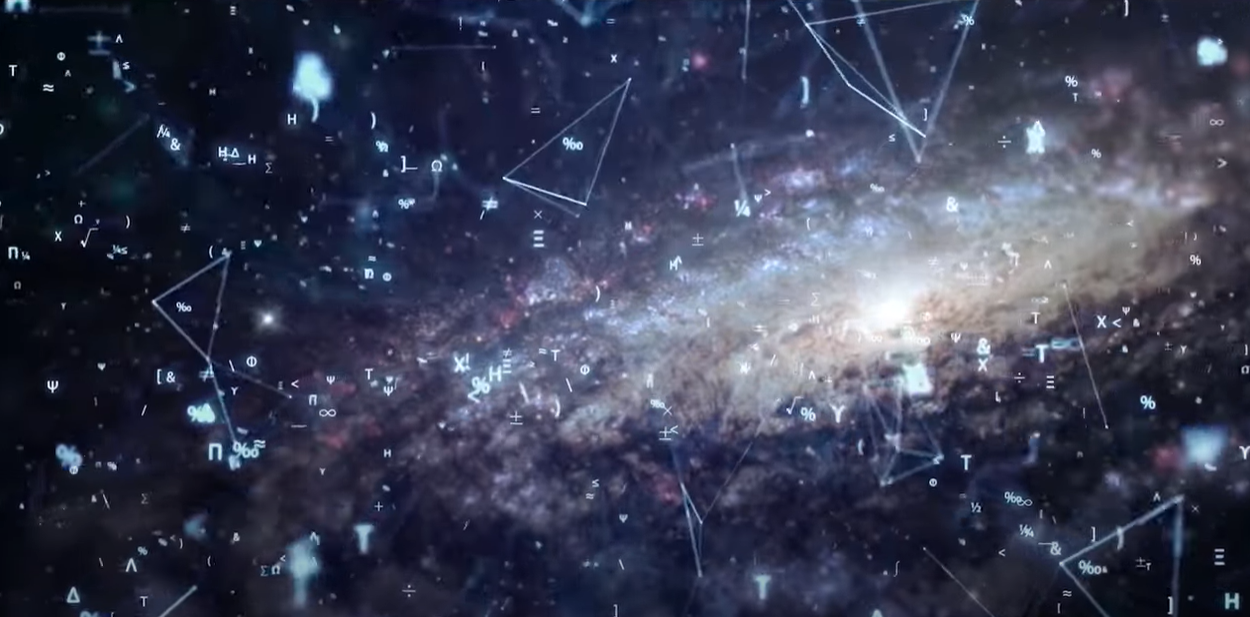We must ask ourselves why the Creator would scaffold the entire perfect system of the Torah on the Names of G-d and the entire nearly 80,000-word narrative based on that One G-d if He was not that One G-d and if He did not want to show us how to get closer to Him metaphysically. The numbers are a language, one that existed cosmically before Hebrew, man’s first conscious language; their job is to communicate. The equations are the grammar of that language and show us relationships, and the pathways we can follow to navigate that cosmos and get closer to the Creator. The various levels of Torah understanding help us to navigate physicality with consciousness; the deepest levels help us to breach that consciousness and transcend the trappings of physicality.

### The Two Census

Everyone familiar with the Torah and Old Testament knows that Moses took two censuses of the men of the Tribes of the Israelites in the desert 40 years apart. And after 40 years the net difference between the 12 Tribes was exactly (603550 – 601730) = 1820 men, which matches perfectly 1 for 1 with the 1820 YHVH (יהוה) in the Torah and in the Path of One.

What most scholars miss it that those 12 Tribes can be divided into two groups of 6, the 6 Tribes with zero net change and the 6 Tribes whose total net change was 1820.  What proves that this was of definitive purposeful design is that the gematria value of the Names of the 6 Tribes whose total change was 1820 is also 1820.

Group #1

 Name Name Gematria 1st census 2nd census Difference Reuven רְאוּבֵן 259 46,500 43,730 (2,770) Gad גָּד 7 45,650 40,500 (5,150) Efraim אֶפְרָיִם 331 40,500 32,500 (8,000) Binyamin בִּנְיָמִן 152 35,400 45,600 10,200 Asher אָשֵׁר 501 41,500 53,400 11,900 Naftali נַפְתָּלִי 570 53,400 45,400 (8,000) 1820 262,950 261,130 (1,820)

Group #2

 Name Name Gematria 1st census 2nd census Difference Shimon שִׁמְעוֹן 466 59,300 22,200 (37,100) Yehuda יְהוּדָה 30 74,600 76,500 1,900 Dan דָּן 54 62,700 64,400 1,700 Yisachar יִשָּׂשכָר 830 54,400 64,300 9,900 Zebulun זְבֻלוּן 95 57,400 60,500 3,100 Manasseh מְנַשֶּׁה 395 32,200 52,700 20,500 1870 340,600 340,600 –

Moreover, in the second group of 6 Tribes, the ones with zero net differential, the sum of the gematria of their 6 Names equals 1870, and not only is 1870 equal to (10 x 187), as in the 187 chapters in the Torah, but when we square the circle of 1870, in other words when we apply the Cosmic Harmonic (1.273) to it, we get (1870 x 1.273) = 2380 = 1/42, right back to the Path of One and the Singularity of 42.

The Cosmic Harmonic is the ratio of the area of a circle inscribed in a square to that square, and thus given the formula A = πr2, the ratio is based on the constant Pi (π) and is inherent in the design of our universe, which we will dive into in an upcoming article. It is also designed into the Torah in manifold ways starting with its 5 quantitative elements—words, verses, letters, rows, and columns—that equal 401,273 or precisely 248,000(φ).

There are a few basic themes built into every aspect of the Torah and redundantly woven throughout its technology so we would not miss them, so we could not mistake them, and so they could focus us on the right paths. In subsequent articles we will explore these in greater and greater depth. It is well known that the Torah is written on 70 levels of Understanding. We will be exploring the deepest of those levels: the ones that explain the nature of our physicality and processes of the cosmos from which it is reflected.

Ezra